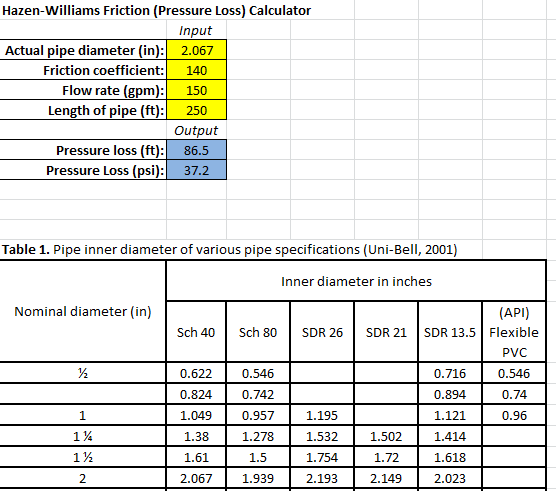# Hazen Williams Excel Sheet Calculator for Head Loss Calculations

Hazen - Williams equation describes the relationship which relates the flow of water in a pipe with the physical properties of the pipe . it can be used to calculate the pressure drop (psi) or friction loss in pipes or tubes .Hazen Williams Excel Sheet

## Hazen Williams Excel Sheet Calculator for Head Loss Calculations

### Hazen Williams Excel Sheet Calculator

Hazen - Williams Calculator excel sheet is a simple tool for obtaining the total head loss in a pipe by entering these parameters:

• Pipe material ( or enter the C value instead )
• Inside diameter
• Flow rate
• Pipe Length
• Fluid Velocity

#### The Hazen Williams software includes solved examples for :

• Hazen williams equation
• Table 1 . Pipe inner diameter of various pipe specifications (Uni-Bell,2001)
• Table 2 . Equivalent Pipe Length of common fittings ( Cidwt, 2008 )